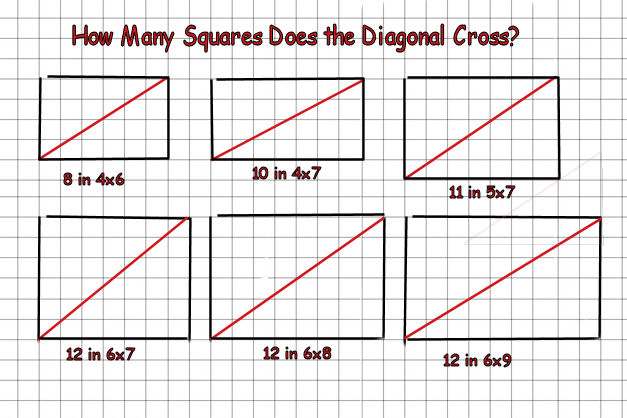# How Many Squares Does the Diagonal Cross ? EditedA diagonal is drawn on the floor from 1 corner to the opposite corner of a rectangular room covered with square tiles 1' x 1'.

A number of tiles are crossed by the diagonal. This depends on the size of the room and the relation of the width and the length.

Let A = # of tiles crossed in a room 32' x 48',

B = # of tiles crossed in a room 32' x 47',

and C = # of tiles crossed in a room 32' x 46'

Find the sum A + B + C

×